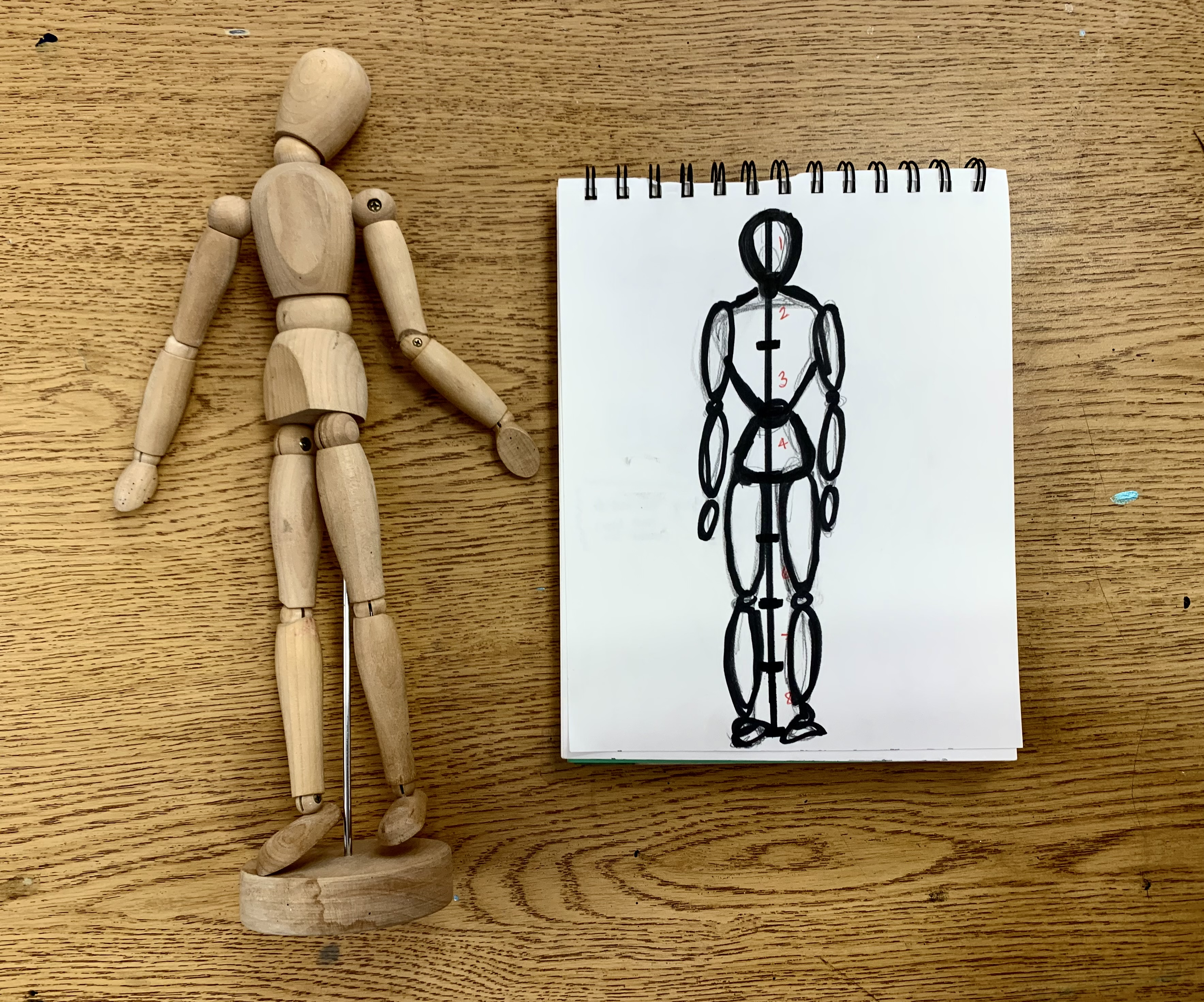# How to Draw Basic Human Proportions

This drawing guide is the most basic of the basic! My students are starting their figure drawing unit and we are started at the very beginning (a very good place to start). We are studying basic human proportions by sketching out a figure using simple shapes and eight even sections.

We used rulers, pencils and sketch paper to draw each simplified body part and where it typically is within an average human body. I always remind students that there are no certainties with real human bodies and this should be used as a guide and not an exact science.

## Figure Sculpting

This is also the same formula I use when students are doing their figure sculptures. My two favorites are expressive clay figures and wire figures! Try out these tutorials here: